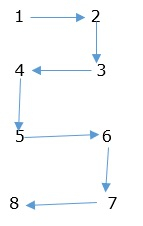# C program to represent the numbers in spiral pattern

The spiral pattern to represent the numbers is shown below −The logic applied to print the numbers in spiral pattern is as follows −

for(i=1;i<=rows*2;i+=2){
if(k%2==1){
printf("%3d %3d",i,i+1);
k++;
}else{
printf("%3d %3d",i+1,i);
k++;
}
printf("");
}

## Program

Following is the C program for representing the numbers in spiral pattern −

#include<stdio.h>
main(){
int i,rows,k=1;
printf("Enter number of Rows for Spiral Pattern");
scanf("%d",&rows);
for(i=1;i<=rows*2;i+=2){
if(k%2==1){
printf("%3d %3d",i,i+1);
k++;
}else{
printf("%3d %3d",i+1,i);
k++;
}
printf("");
}
}

## Output

When the above program is executed, it produces the following result −

Enter number of Rows for Spiral Pattern
10
1 2
4 3
5 6
8 7
9 10
12 11
13 14
16 15
17 18
20 19